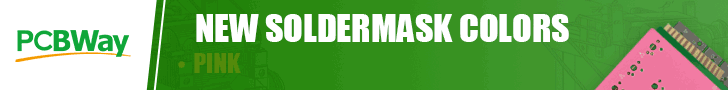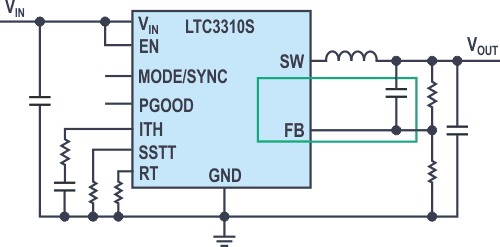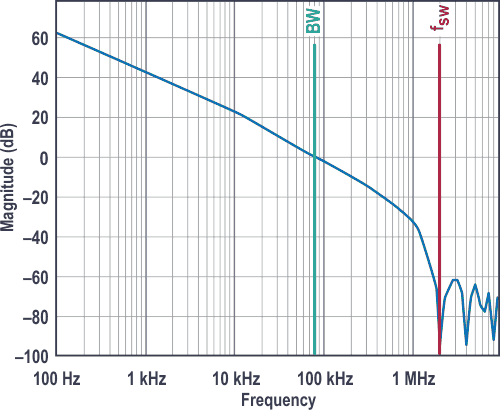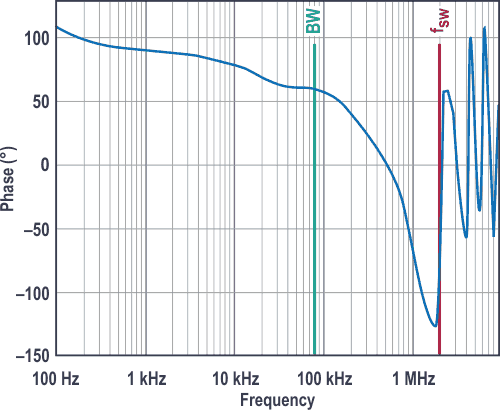# How Bode plots can help you meet the requirements for dynamic control behavior

This article will describe how Bode plots can be used as a tool to quickly assess if your power supply design will meet the requirements for dynamic control behavior. Power supplies normally maintain a fixed output voltage via a control loop. This control loop can be stable or unstable. It can also regulate rapidly or slowly. In most cases, the control loop can be described by means of a Bode plot. With it, you can immediately see how fast or slow the control loop is and especially how stable its regulation.Figure 1 shows a typical switching regulator with a step-down (buck) topology. It converts a higher input voltage, VIN, to a lower output voltage. The goal is to regulate the output voltage, VOUT, as precisely as possible. For this, a control loop is integrated into the circuit through the feedback (FB) pin. It detects voltage changes at VOUT. The control loop should respond quickly so that VOUT is always re-regulated as accurately as possible. The output voltage must be readjusted whenever the input voltage or the load current changes.Figure 1. An example of a switching regulator that uses a control loop (shown in green) to regulate its output voltage.

Figure 2 shows the gain curve for the control loop in a Bode plot. It yields two important pieces of information. The frequency at which the gain is equal to 1 – that is, at 0 dB – can be read. For the control loop in Figure 2, this so-called crossover frequency occurs at approximately 80 kHz. As a rule of thumb, this frequency should be no more than one-tenth of the set switching frequency, fSW, of the switch-mode power supply. Unstable behavior can result if this rule is violated. The second main piece of information shown is the area under the gain curve – that is, the integral of the function. The higher the DC gain and the crossover frequency, the better the control loop can maintain the output voltage at a constant level.Figure 2. A Bode plot showing the gain of the control loop with a 0 dB crossover point at approximately 80 kHz.

Figure 3 shows the phase curve in a Bode plot. The most important value that can be read from this plot is the phase margin. This is a value that provides an indication of the stability of the control loop. The phase margin can be read at the crossover frequency obtained from the gain plot (see Figure 2). In the example shown, the crossover point lies at 80 kHz. Therefore, the phase margin in Figure 3 is approximately 60°. Phase margins of less than approximately 40° are considered unstable. The control loop is optimally set when the phase margin is between 40° and 70°. There is a good compromise between fast regulation and good stability in this range. Above a phase margin of 70°, systems tend to be very stable but with extremely slow regulation.Figure 3. The phase curve for the control loop with a phase margin of 60°.

Bode plots are usually not given in data sheets for switching regulators. This is because the plots depend too strongly on the circuit design. The switching frequency used, the choice of external components – such as the inductor and the output capacitor – and the respective operating conditions – such as input voltage, output voltage, and load current – can have significant effects. For this reason, Bode plots are often generated using computational tools such as LTpowerCAD® or with simulation tools such as LTspice®. With them, you can quickly find out whether a planned circuit can meet the requirements for dynamic control behavior.

Analog Devices